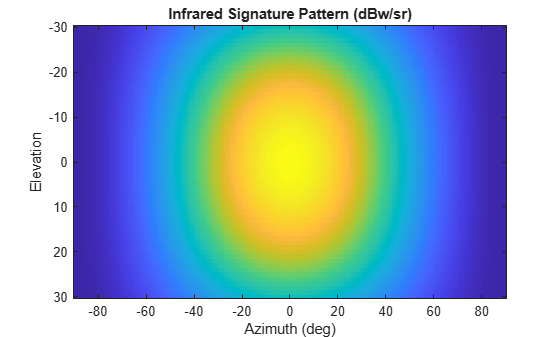# irSignature

Infrared platform signature

## Description

The `irSignature` creates an infrared (IR) signature object. You can use this object to model an angle-dependent contrast radiant intensity of a platform. The radiant intensity is with respect to the background.

## Creation

### Syntax

``irsig = irSignature``
``irsig = irSignature(Name,Value)``

### Description

``` `irsig = irSignature` creates an `irSignature` object with default property values.```

example

````irsig = irSignature(Name,Value)` sets object properties using one or more `Name,Value` pair arguments. `Name` is a property name and `Value` is the corresponding value. `Name` must appear inside single quotes (`''`). You can specify several name-value pair arguments in any order as `Name1,Value1,...,NameN,ValueN`. Any unspecified properties take default values.```

Note

You can only set property values of `irSignature` when constructing the object. The property values are not changeable after construction.

## Properties

expand all

Sampled contrast IR intensity pattern, specified as a scalar, or a Q-by-P real-valued matrix. The pattern is an array of IR values defined on a grid of elevation angles and azimuth angles. Azimuth and elevation are defined in the body frame of the target. Units are dBw/sr.

• Q is the number of IR samples in elevation.

• P is the number of IR samples in azimuth.

Q and P usually match the length of the vectors defined in the `Elevation` and `Azimuth` properties, respectively, with these exceptions:

• If you want to model an IR pattern for an elevation cut (constant azimuth), you can specify the IR pattern as a Q-by-1 vector. Then, the elevation vector specified in the `Elevation` property must have length-2.

• If you want to model an IR pattern for an azimuth cut (constant elevation), you can specify the IR pattern as a 1-by-P vector. Then, the azimuth vector specified in the `Azimuth` property must have length-2.

Example: `[10,0;0,-5]`

Data Types: `double`

Azimuth angles used to define the angular coordinates of each column of the matrix or array specified by the `Pattern` property. Specify the azimuth angles as a length P vector. P must be greater than two. Angle units are in degrees.

Example: `[-45:0.5:45]`

Data Types: `double`

Elevation angles used to define the coordinates of each row of the matrix or array specified by the `Pattern` property. Specify the elevation angles as a length Q vector. Q must be greater than two. Angle units are in degrees.

Example: `[-30:0.5:30]`

Data Types: `double`

Frequencies used to define the applicable IR intensity for each page of the `Pattern` property, specified as a K-element vector of positive scalars. K is the number of IR samples in frequency. K must be no less than two. Frequency units are in hertz.

Example: `[0:0.1:30]`

Data Types: `double`

## Object Functions

 `value` Infrared intensity at specified angle and frequency `toStruct` `irStruct = toStruct(irsig)` converts the `irSignature` object `irsig` to a structure `rcsStruct`. The field names of the returned `irStruct` structure are the same as the property names of the `irsig` object.

## Examples

collapse all

Create and display an IR intensity signature. The signature depends on azimuth and elevation.

Define the azimuth and elevation angle sample points.

```az = -90:90; el = [-30:30];```

Create the IR intensity signature pattern.

```pat = 50*cosd(2*el.')*cosd(az).^2; irsig = irSignature('Pattern',pat,'Azimuth',az,'Elevation',el);```

Display the IR pattern.

```imagesc(irsig.Azimuth,irsig.Elevation,irsig.Pattern) xlabel('Azimuth (deg)') ylabel('Elevation') title('Infrared Signature Pattern (dBw/sr)')```Get the IR intensity value at 25 degrees azimuth and 10 degrees elevation.

`value(irsig,25,10)`
```ans = 38.5929 ```

Get IR intensity value outside of the valid elevation span.

`value(irsig,25,35)`
```ans = -Inf ```